# 4. Content Markup

## 4.4 The Content Markup Elements

This section provides detailed descriptions of the MathML content tags. They are grouped in categories which broadly reflect the area of mathematics from which they come, and also the grouping in the MathML DTD. There is no linguistic difference in MathML between operators and functions. Their separation here and in the DTD is for reasons of clarity.

When working with the content elements, keep in mind the following.

• The role of the content elements is analogous to data entry in a mathematical system. The information that is provided is there to facilitate the successful parsing of an expression as the intended mathematical object by a receiving application.
• MathML content elements do not by themselves "perform" any mathematical evaluations or operations. They do not "evaluate" in a browser and any "action" that is ultimately taken on those objects is determined entirely by the receiving mathematical application. For example, editing programs and applications geared to computation for the lower grades would typically leave 3 + 4  as is, while computational systems targeting a more advanced audience might evaluate this to as 7. Similarly, some computational systems might evaluate sin(0) to 0 while others would leave it unevaluated. Yet other computational systems might be unable to deal with pure symbolic expressions sin(x) and may even regard it as a data entry error. None of this has any bearing on the correctness of the original MathML representation. Where evaluation is mentioned at all in the descriptions below, it is merely to help clarify the meaning of the underlying operation.
• Apart from the instances where there is an explicit interaction with presentation tagging, there is no required rendering (visual or aural) -- only a suggested default. As such, the presentations that are included in this section are merely to help communicate to the reader the intended mathematical meaning by association with the same expression written in a more traditional notation.

The available content elements are:

### 4.4.1 Token Elements

#### 4.4.1.1 <cn>

##### Discussion
The cn element is used to specify actual numerical constants. The content model must provide sufficient information that a number may be entered as data into a computational system. By default, it represents a signed real number in base 10. Thus, the content normally consists of PCDATA restricted to a sign, a string of decimal digits and possibly a decimal point, or alternatively one of the predefined symbolic constants such as π.

The cn element uses the attribute type to represent other types of numbers such as integer, rational, real, complex etc. and base to specify the numerical base.

In addition to simple PCDATA, cn accepts as content PCDATA separated by the <sep/> element. This determines the different parts needed to construct a rational or complex-cartesian number.

Alternative input notations for numbers are possible, but must be explicitly defined by using the definition attribute to refer to a written specification of how a <sep/> separated sequence of real numbers is to be interpreted.

##### Attributes

All attributes are CDATA

• type: real | integer | rational | complex-cartesian |
complex-polar | constant
• base: number (CDATA for XML DTD) between 2 and 36.
• class: CDATA (as per CSS1)
• style: CDATA (as per CSS1)
• definition: CDATA identifying an alternative definition.
Notes:
The type attribute specifies the kind of "number" the cn element's data represents. Each data type implies that the data adheres to certain formating conventions, detailed below. If the data fails to conform to the expected format, an error is generated.

real: A real number is presented in decimal notation. Decimal notation consists of an optional sign ("+" or "-") followed by a string of digits possibly separated into an integer and a fractional part by a "decimal point". Some examples are .3 1 -31.56. If a different BASE is specified, then the digits are interpreted as being digits computed to that base.

A real number may also be presented in scientific notation. Such numbers have two parts (a mantissa and an exponent) separated by e. The first part is a real number while the second part is an integer exponent indicating a power of the base. For example, 12.3e5 represents 12.3 times 10 ^5.

integer: An integer is represented by an optional sign followed by a string of 1 or more "digits". What a "digit" is depends on the base attribute. If base is present, it specifies the base for the digit encoding, and it specifies it base ten. Thus base='16' specifies a hex encoding. When base > 10, letters are added in alphabetical order as digits. The legitimate values for base are therefore between 2 and 36.

rational: A rational number is two integers separated by the <sep/> element. If base is present, it specifies the base used for the digit encoding of both integers.

complex-cartesian: A complex number is of the form two real point numbers separated by <sep/>.

complex-polar: A complex number is specified in the form of a magnitude and an angle (in radians). The raw data is in the form of two real numbers separated by <sep/>.

constant: The "constant" type is used to denote named constants. The data for a constant CN tag must be one of the following common constants:

 Symbol Value π The usual π of trigonometry: approximately 3.141592653... ⅇ The base for natural logarithms: approximately 2.718281828 ... γ Euler's constant: approximately .5772156649...
##### Examples:
<cn type="real"> 12345.7 </cn>
<cn type="integer"> 12345 </cn>
<cn type="integer" base=16> AB3 </cn>
<cn type="rational> 12342 <sep/> 2342342 </cn>
<cn type="complex-cartesian"> 12.3 <sep/> 5 </cn>
<cn type="complex-polar"> 2 <sep/> 3.1415 </cn>
<cn type="constant"> π </cn>
##### Default Renderings

Not all mathematical systems that encounter content based tagging do visual or aural rendering. The receiving applications are free to make use of a number in the manner it normally handles numerical data. Some systems might simplify the rational number 12342/2342342 to 6171/1171171 while pure floating point based systems might approximate this as .5269085385e-2. All numbers might be re-expressed in base 10. The role of MathML is simply to record enough information about the mathematical object and its structure so that it may be properly parsed.

The following renderings of the above MathML expressions are included both to help clarify the meaning of the corresponding MathML encoding and as suggestions for authors of rendering applications. In each case, no mathematical evaluation is intended or implied.

12345.7
12345
AB316
12342 / 2342342
12.3 + 5 i
Polar( 2 , 3.1415 )
π


#### 4.4.1.2 <ci>

##### Discussion

The ci element is used to specify a symbolic name. Such names are used to identify types of mathematical objects. By default they are assumed to represent complex scalars. The ci element may contain arbitrary presentation markup in its content (see chapter 3) so that its presentation as a symbol can be very elaborate.

The ci element uses the type attribute to specify the type of object that it represents. Apart from its default value complex (scalar) valid types include integer, rational, real, complex-cartesian, and more generally, any of the names of the MathML container elements (e.g. vector) or their type values. The definition attribute can be used to extend the definition of ci to include other types. For example, a more advanced use might require a "complex-vector".

##### Examples
1. <ci> x </ci>
2. <ci type="vector"> V </ci>
3. <ci>
<msub>
<mi>x</mi>
<mi>a</mi>
</msub>
</ci>

##### Default Rendering
If the content of a ci element is tagged using presentation tags, that presentation is used. If no such tagging is supplied then the CDATA content would typically be rendered as if it were the content of an mi element. A renderer may wish to make use of the value of the type attribute to improve on this. For example, a symbol of type vector might be rendered using a bold face. Typical renderings of the above symbols are:
1. x
2. V
3. xi


### 4.4.2 Basic Content Elements

#### 4.4.2.1 <apply>

##### Discussion

The apply element allows a function or operator to be applied to its arguments. Nearly all expression construction in MathML content markup is carried out by applying operators or functions to arguments. The first child of apply is the operator, to be applied, with the other child elements as arguments.

The apply element is conceptually necessary in order to distinguish between a function or operator, and an instance of its use. The expression constructed by applying a function to 0 or more arguments is always an element from the range of the function.

Proper usage depends on the operator that is being applied. For example, the plus operator may have zero or more arguments. while the minus operator requires 1 or 2 arguments to be properly formed.

If the object being applied as a function is not already one of the elements known to be a function (such as fn, sin or plus) then it is treated as if it were the contents of an fn element.

Some operators such as diff and int make use of "named" arguments. These special arguments are elements which appear as children of the apply element and identify "parameters" such as the variable of differentiation or the domain of integration.

##### Examples
1. <apply><factorial/>
<cn>3</cn>
</apply>
2. <apply><plus/>
<cn>3</cn>
<cn>4</cn>
</apply>
3. <apply><sin/>
<ci>x</ci>
</apply>

##### Default Rendering

A mathematical system which has been passed an apply element is free to do with it whatever it normally does with such mathematical data. It may be that no rendering is involved (e.g. a syntax validator), or that the "function application" is evaluated and that only the result is rendered (e.g. sin(0) -> 0).

When an unevaluated "function application" is rendered there are a wide variety of appropriate renderings. The choice often depends on the function or operator being applied. Applications of basic operations such as plus are generally presented using an infix notation while applications of sin would use a more traditional functional notation such as sin (x). Consult the default rendering for the operator being applied.

Applications of user defined functions (see fn) which are not evaluated by the receiving or rendering application would typically render using a traditional functional notation unless an alternative presentation is specified using the semantics tag.

#### 4.4.2.2 <relation>

##### Discussion
The relation element is used to construct an equation or relation. Relations are constructed in a manner exactly analogous to the use of apply. A separate construct is used because the manner in which relations are used differs substantially from that of general algebraic expressions.

The first child of relation is the relational operator, to be applied, with the other child elements as arguments.

See the Usage Guide for further details.

##### Examples and Usage
<relation><eq/>
<ci> a </ci>
<ci> b </ci>
</relation>

<relation><lt/>
<ci> a </ci>
<ci> b </ci>
</relation>

##### Default Rendering
a = b
a < b


#### 4.4.2.3 <fn>

##### Discussion
The fn element makes explicit the fact that a more general MathML object is intended to be used in the same manner as if it were a pre-defined function such as sin or plus.

It is the primary mechanism used to extend the collection of "known" mathematical functions. The definition attribute allows for a specific written definition to be referenced through, for example, a URL. (New functions may also be introduced by using a declaration in conjunction with a lambda expression.)

##### Examples
1. <fn><ci> L </ci>
</fn>

2.
<fn definition="http://www.openmath.org/CDs/MyDC/MyDefinition">
<ci>
<msub>
<mi>J</mi>
<mn>0</mn>
</msub>
</ci>
</fn>

3. <fn>
<apply> <plus/>
<ci> f </ci>
<ci> g </ci>
</apply>
</fn>

##### Default Rendering
An fn object is rendered in the same way as its content.
1. L
2. J0
3. f + g


#### 4.4.2.4 <interval>

##### Discussion

The interval element is used to represent simple mathematical intervals of the real number line. It takes an attribute closure which can take on any of the values open, closed, open-closed, or closed-open, with a default value of closed.

More general domains are constructed by using the condition element to bind free variables to constraints.

The interval element expects either two child elements which evaluate to real numbers or one child element which is a condition defining the interval. interval accepts a closure attribute which specifies if the interval is open, closed, or half open.

##### Examples
1. <interval>
<ci> a </ci>
<ci> b </ci>
</interval>

2. <interval closure="open-closed">
<ci> a </ci>
<ci> b </ci>
</interval>

##### Default Rendering
[a,b]
(a,b]


#### 4.4.2.5 <inverse>

##### Discussion

The inverse element is applied to a function in order to construct a generic expression for the functional inverse of that function. (See also the discussion of inverse in section 4.1.3.7). As with other MathML functions, inverse may either be applied to arguments, or it may appear alone, in which case it represents an abstract inversion operator acting on other functions.

A typical use of the inverse element is in an HTML document discussing a number of alternative definitions for a particular function so that there is a need to write and define f (-1)(x).

To associate a particular definition with f  (-1), use the definition attribute.

##### Examples
1. <apply><inverse/>
<ci> f </ci>
</apply>

2. <apply>
<inverse definition="MyDefinition"/>
<ci> f </ci>
</apply>

3. <apply>
<apply><inverse/>
<ci type="matrix"> a </ci>
</apply>
<ci> A </ci>
</apply>

##### Default Rendering
The default rendering for a functional inverse makes use of a parenthesized exponent as in f (-1)(x).

#### 4.4.2.6 <sep/>

##### Discussion
The sep element is to separate CDATA into separate tokens for parsing the contents of the various specialized forms of the cn elements. For example sep is used when specifying the real and imaginary parts of a complex number (see Section 4.4.1). If it occurs between proper elements, it is an error.
##### Examples
<cn type="complex"> 3 <sep/> 4
</cn>

##### Default Rendering
The sep element is not directly rendered.

#### 4.4.2.7 <condition>

##### Discussion
The condition element is used to place a condition on one or more free variables or identifiers. The conditions may be specified in terms of relations that are to be satisfied by the variables, including general relationships such as set membership.

It is used to define general sets and lists in situations where the elements cannot be explicitly enumerated. For example, it is used below to describe the set of all x such that x < 5. See the discussion on sets in section section 4.4.6.

##### Examples
1.  <condition>
<ci> x </ci>
<relation> <lt/>
<ci> x </ci>
<cn> 5 </cn>
</relation>
</condition>

2.  <condition>
<ci> x </ci>
<relation> <in/>
<ci> x </ci>
<ci type="set"> R </ci>
</relation>
</condition>

3.  <condition>
<ci> x </ci>
<ci> y </ci>
<relation><lt/>
<ci> x </ci>
<ci> y </ci>
</relation>
</condition>

##### Default Rendering
1. x | x < 5
2. x | x in R
3. x,y | x < y


#### 4.4.2.8 <declare>

##### Discussion
The declare construct has two primary roles. The first is to change or set the default attribute values for a specific mathematical object. The second is to establish an association between a "name" and an object. Once a declaration is in effect, the "name" object acquires the new attribute settings, and (if the second object is present) all the properties of the associated object.

The various attributes of the declare element assign properties to the object being declared or determine where the declaration is in effect.

By default, the scope of a declaration is "local" to the surrounding container. The scope attribute can be used to extend this. In particular, setting this attribute value to "global" extends the scope of the definition to the entire containing math element. A scope attribute value of "global-document" indicates that the definition is intended for the entire document.

Editorial Note: Global document scoping is important to the convenient interaction with mathematical notation. However, it is expected that a full and proper implementation of scoping to this level will involve interaction between document styles (CSS1) and MathML.
declare takes 1 or 2 children. The first, mandatory, child is a ci containing the identifier being declared.

<declare type="vector"> <ci> V </ci> </declare>


The second, optional, child is a constructor initialising the variable
<declare type="vector">
<ci> V </ci>
<vector>
<cn> 1 </cn><cn> 2 </cn><cn> 3 </cn>
</vector>
</declare>



The constructor type and the type of the element declared must agree. For example, if the type attribute of the declaration is fn, the second child (constructor) must be an element equivalent to an fn element (This would include actual fn elements, lambda elements and any of the defined function in the basic set of content tags.) If no type is specified in the declaration then the type attribute of the declared name is set to the type of the constructor (second child) of the declaration. The type attribute of the declaration can be especially useful in the special case of the second element being a symantic tag,

##### Attributes

All attributes are CDATA

• type - defines the MathML element type of the identifier declared.
• scope - defines the scope of application of the declaration.
• nargs - number of arguments for function declarations.
• occurrence - describes operator usage as "prefix", "infix" or "function-model" indications
• definition - detailed semantics of the function. Typically URL to something like an OpenMath content dictionary that contains the definition.
##### Examples
The declaration
<declare type="fn" nargs="2" scope="local"
definition="URL for CD defining addition in some function space">
<ci> f </ci>
<apply><plus/>
<ci> F </ci><ci> G </ci>
</apply>
</declare>


declares f to be a two-variable function with the property that f(x,y) = (F + G)(x,y).

The declaration

<declare type="fn">
<ci> J </ci>
<lambda><ci> x </ci>
<apply><ln/>
<ci> x </ci>
</apply>
</lambda>
</declare>


associates the name J with a one-variable function defined so that J(x) = ln x (Note that because of the type attribute of the declare element, the second argument must be something of type fn, namely a known function like sin, an fn construct, or a lambda construct.)

The type attribute on the declaration is only necessary if if the type cannot be inferred from the type of the second argument.

Even when a declaration is in effect it is still possible to override attributes values selectively as in <ci type="integer"> V </ci> . This capability is needed in order to write statements of the form "Let S be a member of S".

##### Default Rendering

Since the declare construct is not directly rendered, most declarations are likely to be invisible to a reader. However, declarations can produce quite different effects in an application which evaluates or manipulates MathML content. While the declaration

<declare>
<ci> v </ci>
<vector>
<cn> 1 </cn>
<cn> 2 </cn>
<cn> 3 </cn>
</vector>
</declare>

is active the symbol v acquires all the properties of the vector, and even its dimension and components have meaningful values. This may affect how v is rendered by some applications, as well as how it is treated mathematically.

#### 4.4.2.9 <lambda>

##### Discussion
The lambda element is used to construct a user-defined function from an expression and one or more free variables. The lambda construct with n internal variables takes n + 1 children. The first n children identify the variables which are used as placeholders in the last child for actual parameter values.

See the Usage Guide for further details.

##### Examples
The following markup represents lambda(x, sin x+1).
<lambda>
<ci> x </ci>
<apply><sin/>
<apply><plus/>
<ci> x </ci>
<cn> 1 </cn>
</apply>
</apply>
</lambda>



The following examples constructs a one argument function in which the argument b specifies the upper bound of a specific definite integral.

<lambda/>
<ci> b </ci>
<apply>
<int/>
<apply><fn>f</fn>
<ci> x </ci>
</apply>
<bvar>
<ci> x </ci>
</bvar>
<lowlimit>
<ci> a </ci>
</lowlimit>
<uplimit>
<ci> b </ci>
</uplimit>
</apply>
</lambda/>

Such constructs are often used conjunction with declare to construct new functions.
##### Default Rendering
Lambda(x,f(x))


### 4.4.3 Arithmetic, Algebra and Logic

#### 4.4.3.1 <quotient/>

##### Discussion

The quotient element is the operator used for division modulo a particular base. When the quotient operator is applied to integer arguments a and b, the result is the "quotient of a divided by b". That is, quotient returns the unique integer, q such that a = q b + r. (In common usage, q is called the quotient and r is the remainder.)

The quotient element takes the attribute definition if it is desired to override the default semantics.

The quotient element is a binary arithmetic operator.

See the Usage Guide for further details.

##### Example
<apply><quotient/>
<ci> a </ci>
<ci> b </ci>
</apply>

Various mathematical applications will use this data in different ways. Editing applications might choose an image such as shown below, while a computationally based application would evaluate it to 2 when a = 13 and b = 5.
##### Default Rendering
There is no commonly used notation for this concept. Some possible renderings are
1. quotient of a divided by b
2. integer part of a/b
3.#### 4.4.3.2 <exp/>

##### Discussion

The exp element represents the function associated with the inverse of the ln function. In particular, exp(1) is approximately 2.718281828.

exp takes the attribute definition which may be used to override the default semantics.

The exp element is a unary arithmetic operator.

See the Usage Guide for further details.

##### Example
<apply><exp/>
<ci> x </ci>
</apply>

##### Default Rendering
ex


#### 4.4.3.3 <factorial/>

##### Discussion

The factorial element is used to construct factorials.

The factorial element takes the attribute definition which may be used to override the default semantics.

The factorial element is a unary arithmetic operator.

See the Usage Guide for further details.

##### Example
<apply><factorial/>
<ci> n </ci>
</apply>

If this were evaluated at n = 5 it would evaluate to 120.
##### Default Rendering
n!


#### 4.4.3.4 <divide/>

##### Discussion
The divide element is the division operator.

The divide element takes the attribute definition which may be used to override the default semantics.

The divide element is a binary arithmetic operator. See the Usage Guide for further details.

##### Example
<apply> <divide/>
<ci> a </ci>
<ci> b </ci>
</apply>

As a MathML expresssion, this does not evaluate. However, on receiving such an expression, some applications may attempt to evaluate and simplify the value. For example, when a=5 and b=2 some mathematical applications may evaluate this to 2.5 while others will treat is as a rational number.
##### Default Rendering
a/b


#### 4.4.3.5 <max/> and <min/>

##### Discussion

The elements max and min are used to compare the values of their arguments. They return the maximum and minimum of these values respectively.

The max and min elements take the attribute definition which can be used to override the default semantics.

The max and min elements are nary arithmetic operators. See the Usage Guide for further details.

##### Examples
When the objects are to be compared explicitly they are listed as arguments to the function as in:
<apply><max/>
<ci> a </ci>
<ci> b </ci>
</apply>

The elements to be compared may also be described using a condition element as in:
<apply><min/>
<condition>
<ci>x</ci>
<relation> <notin/>
<ci> x </ci>
<ci type="set"> B </ci>
</relation>
</condition>
</apply>


##### Default Rendering
max {a, b}
min { x | x notin B }


#### 4.4.3.6 <minus/>

##### Discussion

The minus element is the subtraction operator

The minus element takes the attribute definition which can be used to override the default semantics.

The minus element can be used as a unary arithemtic operator (e.g. to represent -x) or as a binary arithmetic operator (e.g. to represent x-y). See the Usage Guide for further details.

##### Example
<apply> <minus/>
<ci> x </ci>
<ci> y </ci>
</apply>

If this were evaluated at x= 5 and y = 2 it would yield 3.
##### Default Rendering
x - y


#### 4.4.3.7 <plus/>

##### Discussion
The plus element is the addition operator.

The plus element takes the attribute definition which can be used to override the default semantics.

The plus element is an nary arithemtic operators. See the Usage Guide for further details.

##### Example
<apply><plus/>
<ci> x </ci>
<ci> y </ci>
<ci> z </ci>
</apply>

If this were evaluated at x = 5, y = 2 and z = 1 it would yield 8.
##### Default Rendering
x + y + z


#### 4.4.3.8 <power/>

##### Discussion
The power element is generic exponentiation operator. That is, when applied to arguments a and b, it returns the value the "a to the power of b".

The power element takes the attribute definition which can be used to override the default semantics.

The power element is an binary arithmetic operators. See the Usage Guide for further details.

##### Example
<apply><power/>
<ci> x </ci>
<cn> 3 </cn>
</apply>

If this were evaluated at x= 5 it would yield 125.
##### Default Rendering
x3


#### 4.4.3.9 <rem/>

##### Discussion

The rem element is the operator which return the "remainder" of a division modulo a particular base. When the rem operator is applied to integer arguments a and b, the result is the "remainder of a divided by b". That is, rem returns the unique integer, r such that a = q b + r, which r < q. (In common usage, q is called the quotient and r is the remainder.)

The rem element takes the attribute definition which can be used to override the default semantics.

The rem element is an binary arithmetic operator. See the Usage Guide for further details.

##### Example
<apply><rem/>
<ci> a </ci>
<ci> b </ci>
</apply>

If this were evaluated at a = 15 and b = 8 it would yield 7.
##### Default Rendering
a mod b


#### 4.4.3.10 <times/>

##### Discussion

The times element is the multiplication operator.

times takes the attribute definition which can be used to override the default semantics.

##### Example
<apply> <times/>
<ci> a </ci>
<ci> b </ci>
</apply>

If this were evaluated at a = 5.5 and b = 3 it would yield 16.5.
##### Default Rendering
a b


#### 4.4.3.11 <root/>

##### Discussion

The root element is used to construct roots. The kind of root to be taken is specified by a degree element, which should be given as the second child of the apply element enclosing the root element. Thus, square roots correspond to the case where degree contains the value 2, cube roots correspond to 3, and so on. If no degree is present, a default value of 2 is used.

The root element takes the attribute definition which can be used to override the default semantics.

The root element is an operator taking qualifiers. See the Usage Guide for further details.

##### Example

The nth root of a is is given by

<apply> <root/>
<ci> a </ci>
<ci> n </ci>
</apply>

##### Default Rendering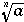#### 4.4.3.12 <gcd/>

##### Discussion

The gcd element is used to denote the greatest common divisor of its arguments.

The gcd takes the attribute definition which can be used to override the default semantics.

The root element is an nary operator. See the Usage Guide for further details.

##### Example
<apply> <gcd/>
<ci> a </ci>
<ci> b </ci>
<ci> c </ci>
</apply>

If this were evaluated at a = 15, b = 21, c = 48 it would yield 3.
##### Default Rendering
GCD(a, b, c)


#### 4.4.3.13 <and/>

##### Discussion

The and element is the boolean "and" operator.

The and element takes the attribute definition which can be used to override the default semantics.

The and element is an nary logical operator. See the Usage Guide for further details.

##### Example
<apply><and/>
<ci> a </ci>
<ci> b </ci>
</apply>

If this were evaluated and both a and b had truth values of "true", then the value would be "true".
##### Default Rendering
a and b


#### 4.4.3.14 <or/>

##### Discussion

The or element is the boolean "or" operator.

The or element takes the attribute definition which can be used to override the default semantics.

The or element is an nary logical operator. See the Usage Guide for further details.

##### Example
<apply><or/>
<ci> a </ci>
<ci> b </ci>
</apply>

##### Default Rendering
a or b


#### 4.4.3.15 <xor/>

##### Discussion

The xor element is the boolean "exclusive or" operator.

xor takes the attribute definition which can be used to override the default semantics.

The xor element is an nary logical operator. See the Usage Guide for further details.

##### Example
<apply><xor/>
<ci> a </ci>
<ci> b </ci>
</apply>

##### Default Rendering
a XOR b


#### 4.4.3.16 <not/>

The not operator is the boolean "not" operator.

The not element takes the attribute definition which can be used to override the default semantics.

The not element is an unary logical operator. See the Usage Guide for further details.

##### Example
<apply><not/>
<ci> a </ci>
</apply>

##### Default Rendering
NOT a


### 4.4.4 Relations

#### 4.4.4.1 <eq/>

##### Discussion

The eq element is the "equals" relational operator.

The eq element takes the attribute definition which can be used to override the default semantics.

The equals element is an nary relation. See the Usage Guide for further details.

##### Example
<relation><eq/>
<ci> a </ci>
<ci> b </ci>
</relation>

If this were tested at a = 5.5 and b = 6 it would yield the truth value "FALSE".
##### Default Rendering
a = b


#### 4.4.4.2 <neq/>

##### Discussion

The neq element is the "not equal to" relational operator.

neq takes the attribute definition which can be used to override the default semantics.

The neq element is an binary relation. See the Usage Guide for further details.

##### Example
<relation><neq/>
<ci> a </ci>
<ci> b </ci>
</relation>

If this were tested at a = 5.5 and b = 6 it would yield the truth value "TRUE".
##### Default Rendering#### 4.4.4.3 <gt/>

##### Discussion
The gt is the "greater than" relational operator.

The gt element takes the attribute definition which can be used to override the default semantics.

The gt element is an nary relation. See the Usage Guide for further details.

##### Example
<relation><gt/>
<ci> a </ci>
<ci> b </ci>
</relation>

If this were tested at a = 5.5 and b = 6 it would yield the truth value "FALSE".
##### Default Rendering
A > B


#### 4.4.4.4 <lt/>

##### Discussion

The lt element is the "less than" relational operator.

The lt element takes the attribute definition which can be used to override the default semantics.

The lt element is an nary relation. See the Usage Guide for further details.

##### Example
<relation><lt/>
<ci> a </ci>
<ci> b </ci>
</relation>

If this were tested at a = 5.5 and b = 6 it would yield the truth value "TRUE".
##### Default Rendering
A < B


#### 4.4.4.5 <geq/>

##### Discussion

The geq is the "greater than or equal" relational operator.

The geq element takes the attribute definition which can be used to override the default semantics.

The geq element is an nary relation. See the Usage Guide for further details.

##### Example
<relation><geq/>
<ci> a </ci>
<ci> b </ci>
</relation>

If this were tested for a = 5.5 and b = 5.5 it would yield the truth value "TRUE".
##### Default Rendering#### 4.4.4.6 <leq/>

##### Discussion

The leq element is the "less than or equal" relational operator.

The leq element takes the attribute definition which can be used to override the default semantics.

The leq element is an nary relation. See the Usage Guide for further details.

##### Example
<relation><lt/>
<ci> a </ci>
<ci> b </ci>
</relation>

If a = 5.4 and b = 5.5 this will yield the truth value "TRUE".
##### Default Rendering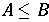#### 4.4.4.7 <implies/>

##### Discussion

The implies element is the boolean "implies" relational operator.

The implies element takes the attribute definition which can be used to override the default semantics.

The implies element is a binary logical operator. See the Usage Guide for further details.

##### Example
<relation><implies/>
<ci> A </ci>
<ci> B </ci>
</relation>

Mathematical applications designed for the evaluation of such expressions would evaluate this to "true" when a = "false" and b = "true". Hoever, such expressions do not evaluate in MathML itsself and would typically not be evaluated by rendering or syntax validation software.
##### Default Rendering### 4.4.5 Calculus

#### 4.4.5.1 <ln>

##### Discussion

The ln element is the natural logarithm operator.

The ln element takes the attribute definition which can be used to override the default semantics.

The ln element is an unary calculus operator. See the Usage Guide for further details.

##### Example
<apply><ln/>
<ci> a </ci>
</apply>

If a = e this will yield the value 1.
##### Default Rendering
ln a


#### 4.4.5.2 <log>

##### Discussion
The log element is the operator which returns a logarithm to a given base. The base may be specified using a logbase element, which should be the second child of the containing apply element. If the logbase element is not present, a default base of 10 is assumed.

The log element takes the attribute definition which can be used to override the default semantics.

The ln element is either an operator taking qualifiers or a unary calculus operator. See the Usage Guide for further details.

##### Example
<apply> <log/>
<ci> x </ci>
<logbase>
<cn> 3 </cn>
</logbase>
</apply>

This markup represents "log x base 3". For natural logarithms base e , the ln element should be used instead.
##### Default Rendering
log3 x


#### 4.4.5.3 <int/>

##### Discussion

The int element is the operator element for an integral. The lower limit, upper limit and bound variable are given by (optional) child elements lowlimit, uplimit and bvar in the enclosing apply element. The integrand is also specified as a child element of the enclosing apply element.

The integration domain may also be specified by using an interval element, or by a condition. In such cases, the bound variable must still be specified since the condition may involve more than one symbol.

The int element takes the attribute definition which can be used to override the default semantics.

The int element an operator taking qualifiers. See the Usage Guide for further details.

##### Examples
<apply>
<int/>
<apply><sin/>
<ci> x </ci>
</apply>
<bvar>
<ci> x </ci>
<bvar>
<lowlimit>
<ci> a </ci>
<lowlimit>
<uplimit>
<ci> b </ci>
<uplimit>
</apply>

<apply><int/>
<apply><cos/>
<ci> x </ci>
</apply>
<bvar>
<ci> x </ci>
<bvar>
<interval>
<ci> a </ci>
<ci> b </ci>
</interval>
</apply>

<apply><int/>
<apply><fn>f</fn>
<ci> x </ci>
</apply>
<bvar>
<ci> x </ci>
<bvar>
<condition>
<ci> x </ci>
<relation>
<ci> x </ci>
<ci> D </ci>
</relation>
</condition>
</apply>

##### Default Rendering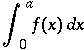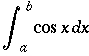#### 4.4.5.4 <diff/>

##### Discussion

The diff element is the differentiation operator element for functions of a single real variable. The bound variable is given by a bvar element which should be a child of the containing apply element.

The diff element takes the attribute definition which can be used to override the default semantics.

The diff element an operator taking qualifiers. See the Usage Guide for further details.

##### Example
<apply><diff/>
<apply><fn> f </fn>
<ci> x </ci>
</apply>
<bvar>
<ci> x </ci>
</bvar>
</apply>

##### Default Rendering#### 4.4.5.5 <partialdiff/>

##### Discussion

The partialdiff element is the partial differentiation operator element for functions of several real variables. The bound variables are given by bvar elements, which should be children of the containing apply element. The bvar elements may also contain a degree element, which specifies the order of the partial derivative to be taken in that variable.

The partialdiff element takes the attribute definition which can be used to override the default semantics.

The partialdiff element an operator taking qualifiers. See the Usage Guide for further details.

##### Example
<apply><partialdiff/>
<apply><fn>f</fn>
<ci> x </ci>
<ci> y </ci>
</apply>
<bvar>
<ci> x </ci>
<degree>
<cn> 2 </cn>
<degree>
</bvar>
<bvar>
<ci> y </ci>
<bvar>
</apply>

##### Default Rendering#### 4.4.5.6 <totaldiff/>

##### Discussion
The totaldiff element is the total differentiation operator element for functions of several real variables. In other words, the result of applying the totaldiff operator is a Jacobian matrix of partial derivatives.

The totaldiff element takes the attribute definition which can be used to override the default semantics.

The totaldiff element is an unary calculus operator. See the Usage Guide for further details.

##### Example
<apply><totaldiff/>
<ci> f </ci>
</apply>

##### Default Rendering
Df


#### 4.4.5.7 <lowlimit>

##### Discussion

The lowlimit element is the container element used to indicate the "lower limit" of an operator using qualifiers. For example, in an integral, it can be used to specify the lower limit of integration. Similarly, it is also used to specify the lower limit of an index for sums and products.

The meaning of the lowlimit element depends on the context it is being used in. For further details about how qualifiers are used in conjunction with operators taking qualifiers, consult the Usage Guide.

##### Example
<apply><int/>
<apply><fn> f </fn>
<ci> x </ci>
</apply>
<bvar>
<ci> x </ci>
<bvar>
<lowlimit>
<ci> a </ci>
<lowlimit>
<uplimit>
<ci> b </ci>
<uplimit>
</apply>


##### Default Rendering

The default rendering of the lowlimit element and its contents depends on the context. In the preceding example, it should be rendered as a subscript to the integral sign: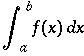Consult the descriptions of individual operators which make use of the lowlimit construct for default renderings.

#### 4.4.5.8 <uplimit>

##### Discussion

The uplimit element is the container element used to indicate the "upper limit" of an operator using qualifiers. For example, in an integral, it can be used to specify the upper limit of integration. Similarly, it is also used to specify the upper limit of an index for sums and products.

The meaning of the lowlimit element depends on the context it is being used in. For further details about how qualifiers are used in conjunction with operators taking qualifiers, consult the Usage Guide.

##### Example
<apply><int/>
<apply><fn> f </fn>
<ci> x </ci>
</apply>
<bvar>
<ci> x </ci>
<bvar>
<lowlimit>
<ci> a </ci>
</lowlimit>
<uplimit>
<ci> b </ci>
</uplimit>
</apply>

##### Default Rendering

The default rendering of the uplimit element and its contents depends on the context. In the preceding example, it should be rendered as a superscript to the integral sign:Consult the descriptions of individual operators which make use of the uplimit construct for default renderings.

#### 4.4.5.9 <bvar>

##### Discussion
The bvar element is the container element for the "bound variable" of an operation. For example, in an integral it specifies the variable of integration. In a derivative, it indicates which variable with respect to which a function is being differentiated. When the bvar element is used to quantifiy a derivative, the bvar element may contain a child degree element which specifies the order of the derivative with respect to that variable. The bvar element is also used for the internal variable in sums and products.

The meaning of the bvar element depends on the context it is being used in. For further details about how qualifiers are used in conjunction with operators taking qualifiers, consult the Usage Guide.

##### Examples
<apply><diff/>
<apply><power/>
<ci> x </ci>
<cn> 2 </cn>
</apply>
<bvar>
<ci> x </ci>
<degree>
<ci> n </ci>
</degree>
</bvar>
</apply>

<apply><int/>
<apply><fn>f</fn>
<ci> x </ci>
</apply>
<bvar>
<ci> x </ci>
</bvar>
<condition>
<ci> x </ci>
<relation>
<ci> x </ci>
<ci> D </ci>
</relation>
</condition>
</apply>

##### Default Rendering

The default rendering of the bvar element and its contents depends on the context. In the preceding examples, it should be rendered as the x in the d x of the integral, and as the x in the denominator of the derivative symbol:Note that in the case of the derivative, the default rendering of the degree child of the bvar element is as an exponent.

Consult the descriptions of individual operators which make use of the uplimit construct for default renderings.

#### 4.4.5.10 <degree>

##### Discussion

The degree element is the container element for the "degree" or "order" of an operation. There are a number basic mathematical constructs which come in families, such as derivatives, moments, and logarithms to various bases. Rather than introduce special elements for each of these families, MathML uses a single general construct, the degree element for this concept of "order".

The meaning of the degree element depends on the context it is being used in. For further details about how qualifiers are used in conjunction with operators taking qualifiers, consult the Usage Guide.

##### Example
<apply><diff/>
<apply><sin/>
<apply> <times/>
<ci> x </ci>
<ci> y </ci>
</apply>
</apply>
<bvar>
<ci> x </ci>
<degree>
<ci> n </ci>
</degree>
</bvar>
<bvar>
<ci> y </ci>
<degree>
<ci> m </ci>
</degree>
</bvar>
</apply>

##### Default Rendering

The default rendering of the degree element and its contents depends on the context. In the preceding example, the degree elements would be rendered as the exponents in the differentiation symbols:Consult the descriptions of individual operators which make use of the uplimit construct for default renderings.

### 4.4.6 Theory of Sets

#### 4.4.6.1 <set>

##### Discussion

The set element is the container element which constructs a set of elements. The elements of a set can be defined either by explicitly listing the elements, or by a condition construction.

The set element is a constructor container. See the Usage Guide for further details.

##### Examples
<set>
<ci> b </ci>
<ci> a </ci>
<ci> c </ci>
</set>

<set>
<condition>
<ci> x </ci>
<relation><lt/>
<ci> x </ci>
<cn> 5 </cn>
</relation>
</condition>
</set>

##### Default Rendering
{a,b,c}

{x | x < 5 }


#### 4.4.6.2 <list>

##### Discussion

The list element is the container element which constructs a list of elements. Elements can be defined either by explicitly listing the elements, or by a condition.

Lists differ from sets in that there is an explicit order to the elements. Two orders are supported: lexicographic and numeric. The kind of ordering which should be used is specified by the order attribute.

The list element is a constructor container. See the Usage Guide for further details.

##### Examples
<list>
<ci> b </ci>
<ci> a </ci>
<ci> c </ci>
</list>

<list order="numeric">
<condition>
<ci> x </ci>
<relation>
<ci> x </ci>
<cn> 5 </cn>
</relation>
</condition>
</list>

##### Default Rendering
[a, b, c]
[x | x < 5 ]


#### 4.4.6.3 <union/>

##### Discussion

The union element is the operator for a set-theoretic union or join of two (or more) sets.

The union attribute takes the attribute definition which can be used to override the default semantics.

The union element is a nary set operator. See the Usage Guide for further details.

##### Example
<apply><union/>
<ci> a </ci>
<ci> b </ci>
</apply>

##### Default Rendering#### 4.4.6.4 <intersect/>

##### Discussion
The intersect element is the operator for the set-theoretic intersection or meet of two (or more) sets.

The intersect element takes the attribute definition which can be used to override the default semantics.

The intersect element is a nary set operator. See the Usage Guide for further details.

##### Example
<apply><intersect/>
<ci type="set"> A </ci>
<ci type="set"> B </ci>
</apply>

##### Default Rendering#### 4.4.6.5 <in/>

##### Discussion

The in element is the relational operator used for a set-theoretic inclusion ("is in" or "is a member of").

The in element takes the attribute definition which can be used to override the default semantics.

The in element is a binary set relation. See the Usage Guide for further details.

##### Example
<relation>
<in/>
<ci> a </ci>
<ci type="set"> A </ci>
</relation>

##### Default Rendering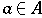#### 4.4.6.6 <notin/>

##### Discussion
The notin element is the relational operator element used for set-theoretic exclusion ("is not in" or "is not a member of").

The notin element takes the attribute definition which can be used to override the default semantics.

The notin element is a binary set relation. See the Usage Guide for further details.

##### Example
<relation><notin/>
<ci> a </ci>
<ci> A </ci>
</relation>

##### Default Rendering#### 4.4.6.7 <subset/>

##### Discussion

The subset element is the realtional operator element for a set-theoretic containment ("is a subset of").

The subset element takes the attribute definition which can be used to override the default semantics.

The subset element is a nary set relation. See the Usage Guide for further details.

##### Example
<relation><subset/>
<ci> A </ci>
<ci> B </ci>
</relation>

##### Default Rendering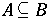#### 4.4.6.8 <prsubset/>

##### Discussion

The prsubset element is the relational operator element for set-theoretic proper containment ("is a proper subset of").

The prsubset element takes the attribute definition which can be used to override the default semantics.

The subset element is a nary set relation. See the Usage Guide for further details.

##### Example
<relation><prsubset/>
<ci> A </ci>
<ci> B </ci>
</relation>

##### Default Rendering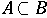#### 4.4.6.9 <notsubset/>

##### Discussion

The notsubset is the relational operator element for the set-theoretic relation "is not a subset of".

The notsubset element takes the attribute definition which can be used to override the default semantics.

The notsubset element is a binary set relation. See the Usage Guide for further details.

##### Example
<relation><notsubset/>
<ci> A </ci>
<ci> B </ci>
</relation>

##### Default Rendering#### 4.4.6.10 <notprsubset/>

##### Discussion

The notprsubset is the operator element for the set-theoretic relation "is not a proper subset of".

The notprsubset takes the attribute definition which can be used to override the default semantics.

The notpresubset element is a binary set relation. See the Usage Guide for further details.

##### Example
<relation><notprsubset/>
<ci> A </ci>
<ci> B </ci>
</relation>

##### Default Rendering#### 4.4.6.11 <setdiff/>

##### Discussion

The setdiff is the operator element for a set-theoretic difference of two sets.

The setdiff element takes the attribute definition which can be used to override the default semantics.

##### Example
setdiff is a binary operator.

The setdiff element is a binary set operator. See the Usage Guide for further details.

<apply><setdiff/>
<ci> A </ci>
<ci> B </ci>
</apply>

##### Default Rendering### 4.4.7 Sequences and Series

#### 4.4.7.1 <sum/>

##### Discussion

The sum element denotes the summation operator. Upper and lower limits for the sum, and more generally a domains for the bound variables are specified using uplimit, lowlimit or a condition on the bound variables. The index for the summation is specified by a bvar element.

The sum element takes the attribute definition which can be used to override the default semantics.

The sum element is an operator taking qualifiers. See the Usage Guide for further details.

##### Examples
<apply><sum/>
<apply><fn>f</fn>
<ci> x </ci>
</apply>
<bvar>
<ci> x </ci>
</bvar>
<lowlimit>
<ci> a </ci>
</lowlimit>
<uplimit>
<ci> b </ci>
</uplimit>
</apply>

<apply><sum/>
<apply><fn>f</fn>
<ci> x </ci>
</apply>
<bvar>
<ci> x </ci>
</bvar>
<condition>
<ci>x</ci>
<relation> <in/>
<ci> x </ci>
<ci type="set"> B </ci>
</relation>
</condition>
</apply>

##### Default Rendering#### 4.4.7.2 <product/>

##### Discussion

The product element denotes the product operator. Upper and lower limits for the product, and more generally a domains for the bound variables are specified using uplimit, lowlimit or a condition on the bound variables. The index for the product is specified by a bvar element.

The product element takes the attribute definition which can be used to override the default semantics.

The product element is an operator taking qualifiers. See the Usage Guide for further details.

##### Examples
<apply><product/>
<apply><fn>f</fn>
<ci> x </ci>
</apply>
<bvar>
<ci> x </ci>
</bvar>
<lowlimit>
<ci> a </ci>
</lowlimit>
<uplimit>
<ci> b </ci>
</uplimit>
</apply>

<apply><product/>
<apply><fn>f</fn>
<ci> x </ci>
</apply>
<bvar>
<ci> x </ci>
<bvar>
<condition>
<ci>x</ci>
<relation> <in/>
<ci> x </ci>
<ci type="set"> B </ci>
</relation>
</condition>
</apply>

Usage Guide
##### Default Rendering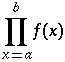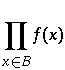#### 4.4.7.3 <limit/>

##### Discussion

The limit element is operation of taking a limit of a sequence. The limit point is expressed by specifying a lowlimit and a bvar or by specifying a condition on one or more bound variables.

The limit element takes the attribute definition which can be used to override the default semantics.

The limit element is an operator taking qualifiers. See the Usage Guide for further details.

##### Examples
<apply><limit/>
<apply><sin/>
<ci> x </ci>
</apply>
<lowlimit>
<cn> 0 </cn>
</lowlimit>
<bvar>
<ci> x </ci>
</bvar>
</apply>

<apply><limit/>
<apply><sin/>
<ci> x </ci>
</apply>
<condition>
<ci> x </ci>
<relation>
<tendsto type="above"/>
<ci> x </ci>
<ci> a </ci>
</relation>
</condition>
<bvar>
<ci> x </ci>
</bvar>
</apply>

##### Default Rendering#### 4.4.7.4 <tendsto/>

##### Discussion

The tendsto element is used to express the relation that a quantity is tending to a specified value.

The tendsto element takes the attributes type to set the direction from which the the limiting value is approached and definition which can be used to override the default semantics.

The tendsto element is a binary relational operator. See the Usage Guide for further details.

##### Examples
<relation>
<tendsto type="above"/>
<apply><power/>
<ci> x </ci>
<cn> 2 </cn>
</apply>
<apply><power/>
<ci> a </ci>
<cn> 2 </cn>
</apply>
</relation>


To express (x, y) -> (f(x, y), g(x, y)), one might use vectors, as in:

<relation>
<tendsto/>
<vector>
<ci> x </ci>
<ci> y </ci>
</vector>
<vector>
<apply><fn>f</fn>
<ci> x </ci>
<ci> y </ci>
</apply>
<apply><fn>g</fn>
<ci> x </ci>
<ci> y </ci>
</apply>
</vector>
</relation>

##### Default Rendering

x2 -> a2

(x, y) -> (f(x, y), g(x, y))


### 4.4.8 Trigonometry

The names of the common trigonometric functions supported by MathML are listed below. Since their standard intepretations are widely known, they are discussed as a group.



##### Discussion

These operator elements denote the standard trigonometrical functions.

These elements all take the attribute definition which can be used to override the default semantics.

They are all unary trigonometric operators. See the Usage Guide for further details.

##### Examples
<apply><sin/>
<ci> x </ci>
</apply>

<apply><sin/>
<apply><plus/>
<apply><cos/>
<ci> x </ci>
</apply>
<apply><power/>
<ci> x </ci>
<cn> 3 </cn>
</apply>
</apply>
</apply>

##### Default Rendering
sin x
sin(cos x + x3)


### 4.4.9 Statistics

#### 4.4.9.1 <mean>

##### Discussion
mean is the operator element for a mean or average.

mean takes the attribute definition which can be used to override the default semantics.

##### Example
mean is an nary operator.
<apply><mean/>
<ci> X </ci>
</apply>

Usage Guide
##### Default Rendering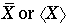#### 4.4.9.2 <sdev>

##### Discussion
sdev is the operator element for the standard deviation.

sdev takes the attribute definition which can be used to override the default semantics.

##### Example
sdev is an nary operator.
<apply><sdev/>
<ci> X </ci>
</apply>

Usage Guide
##### Default Rendering#### 4.4.9.3 <var>

##### Discussion
var is the operator element for the statistical variance.

var takes the attribute definition which can be used to override the default semantics.

##### Example
var is an nary operator.
<apply><var/>
<ci> X </ci>
</apply>

Usage Guide
##### Default Rendering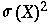#### 4.4.9.4 <median>

##### Discussion
median is the operator element for the median .

median takes the attribute definition which can be used to override the default semantics.

##### Example
median is an nary operator.
<apply><median/>
<ci> X </ci>
</apply>

Usage Guide
##### Default Rendering
median(X)


#### 4.4.9.5 <mode>

##### Discussion
mode is the operator for the statistical mode.

mode takes the attribute definition which can be used to override the default semantics.

##### Example
mode is an nary operator.
<apply>
<mode/>
<ci> X </ci>
</apply>

Usage Guide
##### Default Rendering
mode(X)


#### 4.4.9.6 <moment>

##### Discussion
The moment element represents statistical moments. Use degree for the n in "nth moment".

quotient takes the attribute definition which can be used to override the default semantics.

##### Example
moment is an operator taking qualifiers.
<apply><moment/>
<ci> X </ci>
<degree>
<cn> 3 </cn>
</degree>
</apply>

Usage Guide
##### Default Rendering### 4.4.10 Linear Algebra

#### 4.4.10.1 <vector>

##### Discussion
vector is the container element for a vector. The child elements form the components of the vector.

For purposes of interaction with matrices and matrix multiplication vectors are regarded as equivalent to a matrix consisting of a single column and the transpose of a vector behaves the same as a matrix consisting of a single row.

##### Example
vector is a constructor element.
<vector>
<cn> 1 </cn>
<cn> 2 </cn>
<cn> 3 </cn>
</vector>

Usage Guide
##### Default Rendering
(1,2,3,x)


#### 4.4.10.2 <matrix>

##### Discussion
The matrix is the container element for matrixrow's. matrixrow's contain the elements of a matrix.
##### Example
matrix is a constructor element.
<matrix>
<matrixrow>
<cn> 1 </cn> <cn> 2 </cn>
</matrixrow>
<matrixrow>
<cn> 3 </cn> <ci> x </ci>
</matrixrow>
</matrix>

Usage Guide
##### Default Rendering#### 4.4.10.3 <matrixrow>

##### Discussion
The matrixrow element is the container element for the rows of a matrix.
##### Example
matrixrow is a constructor element.
<matrixrow>
<cn> 1 </cn>
<cn> 2 </cn>
</matrixrow>
<matrixrow>
<cn> 3 </cn>
<ci> x </ci>
</matrixrow>

Usage Guide
##### Default Rendering
Matrix rows are not directly rendered by themselves outside of the context of a matrix.

#### 4.4.10.4 <determinant>

##### Discussion
The determinant element is the operator for constructing the determinant of a matrix.

determinant takes the attribute definition which can be used to override the default semantics.

##### Example
determinant is an unary operator.
<apply><determinant/>
<ci type="matrix"> A </ci>
</apply>

Usage Guide
##### Default Rendering
det A


#### 4.4.10.5 <transpose>

##### Discussion
The transpose element is the operator for constructing the transpose of a matrix.

transpose takes the attribute definition which can be used to override the default semantics.

##### Example
transpose is an unary operator.
<apply><transpose/>
<ci type="matrix"> A </ci>
</apply>

Usage Guide
##### Default Rendering
A-1


#### 4.4.10.6 <select>

##### Discussion
The select element is the operator for indexing into vectors and matrices. It accepts one or more arguments. The first argument identifies the vector or matrix from which the selection is taking place. The second and subsequent arguments indicate the kind of selection taking place. The second argument indicates a vector element, or the row in the case of a matrix. The third argument indicates a position in a matrix row.

When a matrix and only one index i is specified as in

<apply><select>
<matrix>
<matrixrow>
<cn> 1 </cn> <cn> 2 </cn>
</matrixrow>
<matrixrow>
<cn> 3 </cn> <cn> 4 </cn>
</matrixrow>
</matrix>
<cn> 1 </cn>
</apply>

it refers to ith matrixrow. Thus, the preceding example selects the following row:
<matrixrow> <cn> 1 </cn> <cn> 2 </cn> </matrixrow>


select takes the attribute definition which can be used to override the default semantics.

select is an nary linear algebra operator.

##### Example
<apply><select/>
<ci type="matrix"> A </ci>
<cn> 3 </cn>
<cn> 2 </cn>
</apply>

##### Default Rendering
The select construct renders the same as the expression which is selects.

### 4.4.11 Semantic Mapping Elements

The use of the semantic mapping elements is explained in section 4.4.6

#### 4.4.11.1 <annotation>

##### Discussion
The annotation element is the container element for a semantic annotation in a non-XML format.

annotation takes the attribute encoding to define the encoding being used.

##### Example
annotation is a semantic mapping element. It is always used with semantics.
<semantics>

<apply><plus/>
<apply><sin/>
<ci> x </ci>
</apply>
<cn> 5 </cn>
</apply>

<annotation encoding="TeX">
\sin x + 5
</annotation>

</semantics>

None

#### 4.4.11.2 <semantics>

##### Discussion
The semantics element is the container element which associates a MathML construct with alternative represenations. There may be several representations for each object.

The representations which are XML based are enclosed in an xml-annotation element while those representations which are to be parsed as PCDATA are enclosed in an annotation element.

semantics takes the attribute definition which can be used to reference an external source for some or all of the semantic information.

An important purpose of the semantics construct is to associate specific semantics with a particular presentation. When the MathML-presentation annotation is provided, then it is the default presentation. If not, then the default renderings for the associated content expression are used.

##### Examples and Usage
semantics is a semantic mapping element.
<semantics>
<apply><plus/>
<apply><sin/>
<ci> x </ci>
</apply>
<cn> 5 </cn>
</apply>

<annotation encoding="Maple">
sin(x) + 5
</annotation>

<xml-annotation encoding="MathML-Presentation">
...
...
</xml-annotation>

<annotation encoding="Mathematica">
Sin[x] + 5
</annotation>

<annotation encoding="TeX">
\sin x + 5
</annotation>

<xml-annotation encoding="OpenMath">
<OM_APP>..</OM_APP>
</xml-annotation>

</semantics>

None

#### 4.4.11.3 <xml-annotation>

##### Discussion
The xml-annotation container element is used to contain representations that are XML based. It is always used together with

xml-annotation takes the attribute encoding to define the encoding being used. semantics .

##### Example
xml-annotation is a semantic mapping element.
<semantics>

<apply><plus/>
<apply><sin/>
<ci> x </ci>
</apply>
<cn> 5 </cn>
</apply>

<annotation encoding="TeX">
\sin x + 5
</annotation>

</semantics>